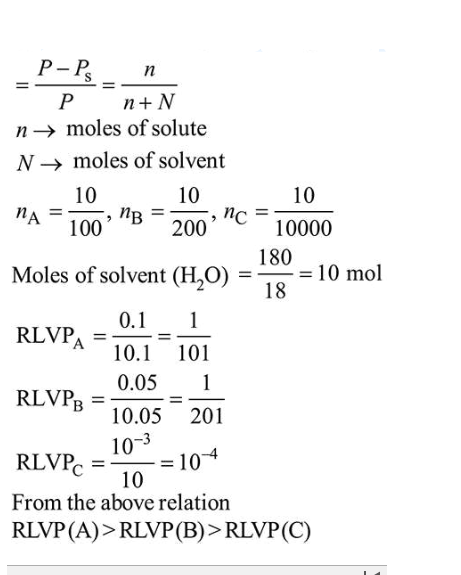# A set of solutions is prepared using 180 g of water as a solvent and 10g of different non-volatile solutes A, B and C.Question:

A set of solutions is prepared using $180 \mathrm{~g}$ of water as a solvent and $10 \mathrm{~g}$ of different non-volatile solutes A, B and C. The relative lowering of vapour pressure in the presence of these solutes are in the order

[Given, molar mass of $\mathrm{A}=100 \mathrm{~g} \mathrm{~mol}^{-1} ; \mathrm{B}=200 \mathrm{~g} \mathrm{~mol}^{-1} ; \mathrm{C}=10,000 \mathrm{~g} \mathrm{~mol}^{-1}$ ]

1.  $\mathrm{B}>\mathrm{C}>\mathrm{A}$

2.  $\mathrm{C}>\mathrm{B}>\mathrm{A}$

3. $\mathrm{A}>\mathrm{B}>\mathrm{C}$

4. $\mathrm{A}>\mathrm{C}>\mathrm{B}$

Correct Option: , 3

Solution:

Relative lowering in vapour pressure (RLVP)